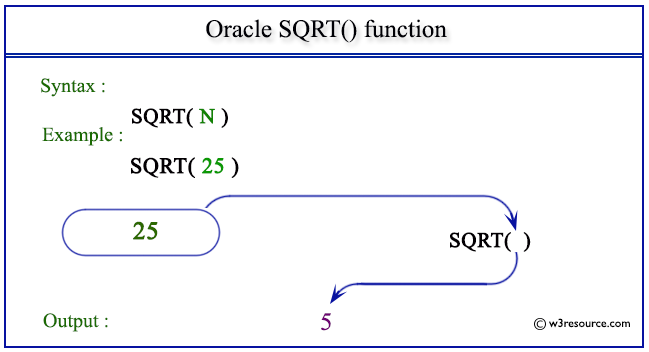# Oracle SQRT() function

## Description

This function is used to get the square root of a given non-negative number.

Syntax:

`SQRT(X)`

Where X is a number

Pictorial Presentation of SQRT() functionExample: SQRT() function

``````SELECT SQRT(25) FROM dual;
```
```

Here is the result.

```  SQRT(25)
----------
5
```

The above statement will return the square root of the given number 25.

Example: SQRT() function using negative value

``````SELECT SQRT(-25) FROM dual;
```
```

Here is the result.

```SELECT SQRT(-25) FROM dual
*
ERROR at line 1:
ORA-01428: argument '-25' is out of range
```

The above statement will return an error message because the value of the argument (-25) is negative.

Previous: SINH
Next: TAN

﻿